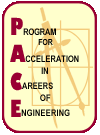# Calculus

This course will train you to think logically; to examine Area and Tangent concepts; and to learn to write the solutions of the problems in an organized manner. Topics will be presented geometrically, numerically, algebraically and descriptively.

Calculus is considered to be one of the greatest achievements of human intelligence. The origins of calculus go back at least 2,500 years to the ancient Greeks. They developed techniques and methods to solve the Area problem, and it was called, Integral Calculus.

Then, 2,000 years later, French mathematicians developed techniques and methods to solve the Tangent problem, and it was called, Differential Calculus.

The two branches of calculus, Integral Calculus and Differential Calculus, and their major problems, the Area problem and the Tangent problem, are inverse problems. In the same way, the Division is the inverse of the Multiplication problem.

The PACE Calculus Class includes topics such as:

1. Functions
2. Limits and its Applications
3. Derivatives
4. Review of Trigonometry as well as the Exponential and the Logarithmic Functions
5. Applications of the Derivative (Physics, Engineering, Biology, Chemistry, Economics)
6. Integrals
7. Applications of Integrals (Physics, Engineering, Biology, Chemistry, Economics)

## Who It’s For

This class is for students that have completed Algebra I, Geometry, Algebra II, Trigonometry, and Pre-Calculus.

## Quizzes

Quizzes are provided here for the student to use for reviewing material throughout the school year and for those students who miss classes to keep abreast of which topic the class has covered.

September 24, 2005

October 1, 2005

October 22, 2005 (functions)

October 22, 2005 (limits)

October 22, 2005 (Calc II)

November 19, 2005 (Differentiation Concepts)

January 21, 2006 (Bio, Physics, Eng, & Chem Derivative Problems)

January 21, 2006 (Critical Points - Ladder Problem)

January 28, 2006 (Integration Concepts)

January 28, 2006 (Applications of Integral - Area)

March 4, 2006 (Integration by Substitution and Integration by Parts)

## Calculus Projects

Calculus Project Presentations